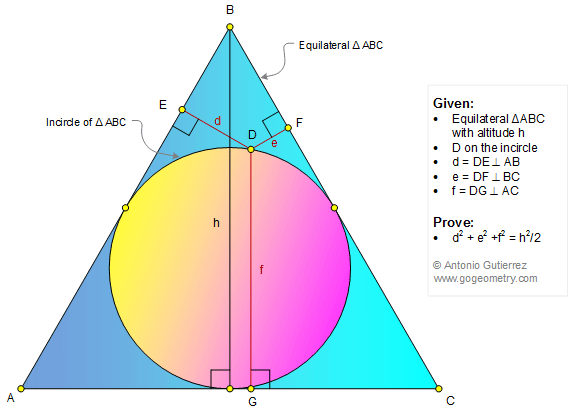# Math Infographic, Geometry Problem 1346: Equilateral Triangle, Point on the Incircle, Altitude, Perpendicular, Sum of Squares, Distance

### Proposition

The figure shows an equilateral triangle ABC with altitude h and a point D on the incircle. DE= d, DF = e, and DG = f are perpendicular  to AB, BC, and AC, respectively. Prove that d2 + e2 + f2 = h2/2.